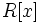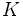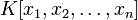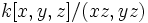# Cohen-Macaulay ring

This article is about a definition in group theory that is standard among the commutative algebra community (or sub-community that dabbles in such things) but is not very basic or common for people outside

View a list of other standard non-basic definitions
This article defines a property of commutative unital rings; a property that can be evaluated for a commutative unital ring
View all properties of commutative unital rings
VIEW RELATED: Commutative unital ring property implications | Commutative unital ring property non-implications |Commutative unital ring metaproperty satisfactions | Commutative unital ring metaproperty dissatisfactions | Commutative unital ring property satisfactions | Commutative unital ring property dissatisfactions

## Definition

### Symbol-free definition

A commutative unital ring is termed Cohen-Macaulay if it is Noetherian and satisfies the following equivalent conditions:

### Equivalence of definitions

Further information: Equivalence of definitions of Cohen-Macaulay

## Metaproperties

Metaproperty name Satisfied? Proof Statement with symbols
polynomial-closed property of commutative unital rings Yes Cohen-Macaulay is polynomial-closed Suppose$R$ is a Cohen-Macaulay ring. Then, the polynomial ring$R[x]$ is also a Cohen-Macaulay ring.
strongly local property of commutative unital rings Yes Cohen-Macaulay is strongly local The following are eqiuvalent for a commutative unital ring$R$: (i)$R$ is Cohen-Macaulay, (ii) the localization at any prime ideal of$R$ is Cohen-Macaulay, (iii) the localization at any maximal ideal of$R$ is Cohen-Macaulay.
quotient-closed property of commutative unital rings No Cohen-Macaulay is not quotient-closed It is possible to have a Cohen-Macaulay ring$R$ and an ideal$I$ in$R$ such that$R/I$ is not Cohen-Macaulay. However, if$I$ is generated by a regular sequence, then the quotient is a Cohen-Macaulay ring. Thus, for instance, the quotient by a principal ideal generated by an element which is not a zero divisor is again a Cohen-Macaulay ring.
finite direct product-closed property of commutative unital rings Yes Cohen-Macaulay is finite direct product-closed Suppose$R_1,R_2,\dots,R_n$ are all Cohen-Macaulay rings. Then, the external direct product$R_1 \times R_2 \times \dots \times R_n$ is also a Cohen-Macaulay ring.

## Relation with other properties

### Conjunction with other properties

Conjunction Other component of conjunction Comments
Cohen-Macaulay domain integral domain
local Cohen-Macaulay ring local ring

### Stronger properties

Property Meaning Proof of implication Proof of strictness (reverse implication failure) Intermediate notions
Regular local ring regular local implies Cohen-Macaulay Cohen-Macaulay not implies regular local click here
regular ring its localization at any prime ideal is a regular local ring Regular implies Cohen-Macaulay Cohen-Macaulay not implies regular click here
polynomial ring over a field has the form$K[x]$ where$K$ is a field click here
multivariate polynomial ring over a field has the form$K[x_1,x_2,\dots,x_n]$ where$K$ is a field click here
principal ideal domain every ideal is a principal ideal, i.e., it is generated by one element click here
Artinian ring every descending chain of ideals stabilizes at a finite length Artinian implies Cohen-Macaulay click here
one-dimensional Noetherian domain one-dimensional Noetherian integral domain One-dimensional Noetherian domain implies Cohen-Macaulay click here

### Weaker properties

Property Meaning Proof of implication Proof of strictness (reverse implication failure) Intermediate notions
universally catenary ring Cohen-Macaulay implies universally catenary universally catenary not implies Cohen-Macaulay click here
This rules out, for instance, rings like$k[x,y,z]/(xz,yz)$.
• The spectrum need not be irreducible: The ring$k[x,y]/(xy)$ is Cohen-Macaulay, although its spectrum has two irreducible components.